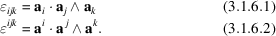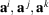International
Tables for
Crystallography
Volume B
Reciprocal space
Edited by U. Shumeli

International Tables for Crystallography (2010). Vol. B, ch. 3.1, p. 405   | 1 | 2 |

Section 3.1.6. Permutation tensors

D. E. Sandsa*

aDepartment of Chemistry, University of Kentucky, Chemistry–Physics Building, Lexington, Kentucky 40506–0055, USA
Correspondence e-mail: sands@pop.uky.edu

3.1.6. Permutation tensors

| top | pdf |

Many relationships involving vector products may be expressed compactly and conveniently in terms of the permutation tensors, defined asSincerepresents the volume of the parallelepiped defined by vectors, it follows thatvanishes if any two indices are equal to each other. The same argument applies, of course, to. That is,If the indices are all different,for even permutations of ijk (123, 231, or 312), andfor odd permutations (132, 213, or 321). Here,for right-handed axes,for left-handed axes, V is the unit-cell volume, andis the volume of the reciprocal cell defined by the reciprocal basis vectors.

A discussion of the properties of the permutation tensors may be found in Sands (1982a). In right-handed Cartesian systems, where, and, the permutation tensors are equivalent to the permutation symbols denoted by.

References

Sands, D. E. (1982a). Vectors and Tensors in Crystallography. Reading: Addison Wesley. Reprinted (1995) Dover Publications.